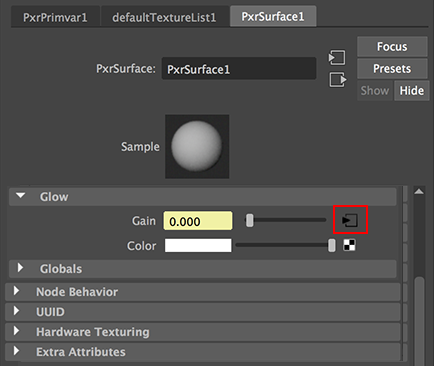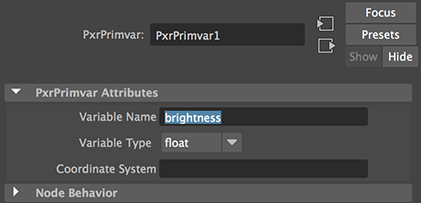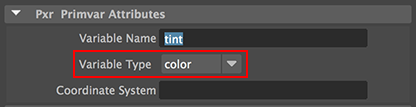### RfMUsing Primvars

##### Related Tutorials:

RIB: Introduction to Primvars
RfM: Multiple Textures
RfM: WyvillEdges

Some useful mel procs for adding primvars can be found in RfM: Customizing.

#### Introduction

This tutorial provides two examples of how primvars can be set up and used with RIS materials. The reader should refer to the tutorial " RIB: Using PrimVars" for basic information about primvars.

To use a primvar with a RIS material, such as PxrSurface, requires both the material and the geometry to which it is assigned to be set up correctly. The first example shows how a single instance of PxrSurface and a float primvar can be used to control the incandescence of many objects. The second example demonstrates how a color primvar can be to used the control the coloration of many objects.

#### Example 1 - using a "float" primvar

##### Setting Up the Material

1     Assign an instance of PxrSurface to several objects.
2     Click the connection icon of the "Gain" input in the "Glow" panel - figure 1.
3     From the list of RIS patterns connect PxrPrimvar.
4     Enter a name of the primvar - figure 2.Figure 1Figure 2

##### Setting Up the Surfaces

5     Copy and paste the MEL proc, shown in listing 1, into Maya's script window.
Execute the script in order to load it into memory.

Listing 1

 ```global proc addConstFloatPrimVar(string \$shortname, float \$minval, float \$maxval) { string \$sel[] = `ls -sl`; string \$fullname = "rmancF" + \$shortname; for(\$current in \$sel) { string \$shapes[] = `listRelatives -s \$current`; string \$shape = \$shapes; if(attributeExists(\$fullname, \$shape) == 0) { addAttr -ln \$fullname -sn \$fullname -k 1 \$shape; } \$value = rand(\$minval, \$maxval); setAttr (\$shape + "." + \$fullname) \$value; } }```

 6     Select the surfaces to which PxrSurface was previously assigned.       Execute the following command,           `addConstFloatPrimVar`("brightness", 0.3, 0.5); The Mel proc will add an attribute named "rmancFbrightness" to the selected objects with random values between 0.3 and 0.5. The name of the attribute consists of the prefix "rmancF" and the name given to the primvar in step 6. When RfM outputs the rib data it will "look" for custom attributes beginning with "rmancF". The prefix tells RfM to add a primvar, declared as a "constant float", to each surface. For example, ```PointsGeneralPolygons   [0 1 3 2] "P" [xyz data...] "constant float brightness" [0.4523]``` 7     Finally, render the scene.Figure 3

#### Example 2 - using a "color" primvar

##### Setting Up the Material

Follow steps 1 to 4 but connect another instance of PxrPrimvar to the Glow "Color" of PxrSurface. Change the variable type and variable name as shown below.Figure 4

##### Setting Up the Surfaces

Copy and paste the MEL proc, shown in listing 2, into Maya's script window.
Execute the script in order to load it into memory.

Listing 2

 ```global proc addConstColorPrimVar(string \$shortname, vector \$minval, vector \$maxval) { string \$sel[] = `ls -sl`; string \$fullname = "rmanC" + \$shortname; for(\$current in \$sel) { string \$shapes[] = `listRelatives -s \$current`; string \$shape = \$shapes; if(size(\$shape) == 0) continue; string \$r_attr = \$shortname +"_r"; string \$g_attr = \$shortname +"_g"; string \$b_attr = \$shortname +"_b"; if(attributeExists(\$fullname, \$shape) == 0) { addAttr -ln \$fullname -nn \$fullname -at "float3" -usedAsColor -k 1 \$shape; addAttr -ln \$r_attr -at "float" -k 1 -parent \$fullname \$shape; addAttr -ln \$g_attr -at "float" -k 1 -parent \$fullname \$shape; addAttr -ln \$b_attr -at "float" -k 1 -parent \$fullname \$shape; } vector \$rgb = rand(\$minval, \$maxval); float \$r = \$rgb.x; float \$g = \$rgb.y; float \$b = \$rgb.z; setAttr (\$shape + "." + \$fullname) -type "float3" \$r \$g \$b; } }```

 Select the surfaces to which PxrSurface was assigned. Execute the following command,     `addConstColorPrimVar("tint", <<0.6, 0.6, 0.6>>, <<1,1,1>>);` The procedure will add an attribute named "rmanCtint" to each of the selected objects with random rgb values of 0.6 and 1.0. In the Rib file "tint" will be declared as a "constant color". For example, ```PointsGeneralPolygons   [0 1 3 2] "P" [xyz data...] "constant color tint" [0.520171 0.486881 0.625127]``` Finally, render the scene.Figure 5